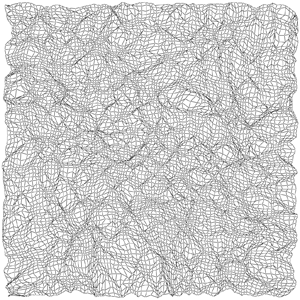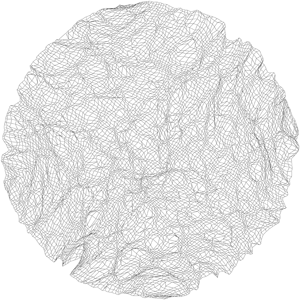#Boost C++ Libraries

...one of the most highly regarded and expertly designed C++ library projects in the world.

This is the documentation for an old version of Boost. Click here to view this page for the latest version.gursoy_atun_layout

### Synopsis

```// Non-named parameter version
template<typename VertexListAndIncidenceGraph,  typename Topology,
typename PositionMap, typename VertexIndexMap,
typename EdgeWeightMap>
void
gursoy_atun_layout(const VertexListAndIncidenceGraph& g,
const Topology& space,
PositionMap position,
int nsteps = num_vertices(g),
double diameter_initial = sqrt((double)num_vertices(g)),
double diameter_final = 1,
double learning_constant_initial = 0.8,
double learning_constant_final = 0.2,
VertexIndexMap vertex_index_map = get(vertex_index, g),
EdgeWeightMap weight = dummy_property_map());

// Named parameter version
template<typename VertexListAndIncidenceGraph, typename Topology,
typename PositionMap, typename P, typename T, typename R>
void
gursoy_atun_layout(const VertexListAndIncidenceGraph& g,
const Topology& space,
PositionMap position,
const bgl_named_params<P,T,R>& params = all defaults);

// Topologies
template<std::size_t Dims> class convex_topology;
template<std::size_t Dims, typename RandomNumberGenerator = minstd_rand> class hypercube_topology;
template<typename RandomNumberGenerator = minstd_rand> class square_topology;
template<typename RandomNumberGenerator = minstd_rand> class cube_topology;
template<std::size_t Dims, typename RandomNumberGenerator = minstd_rand> class ball_topology;
template<typename RandomNumberGenerator = minstd_rand> class circle_topology;
template<typename RandomNumberGenerator = minstd_rand> class sphere_topology;
template<typename RandomNumberGenerator = minstd_rand> class heart_topology;
```

### Description

This algorithm  performs layout of directed graphs, either weighted or unweighted. This algorithm is very different from the Kamada-Kawai and Fruchterman-Reingold algorithms, because it does not explicitly strive to layout graphs in a visually pleasing manner. Instead, it attempts to distribute the vertices uniformly within a topology (e.g., rectangle, sphere, heart shape), keeping vertices close to their neighbors. The algorithm itself is based on Self-Organizing Maps.

Various topologies are provided that produce different, interesting results. The square topology can be used for normal display of graphs or distributing vertices for parallel computation on a process array, for instance. Other topologies, such as the sphere topology (or N-dimensional ball topology) make sense for different problems, whereas the heart topology is just plain fun. One can also define a topology to suit other particular needs.### Where Defined

boost/graph/gursoy_atun_layout.hpp

### Parameters

IN: const Graph& g
The graph object on which the algorithm will be applied. The type Graph must be a model of Vertex List Graph and Incidence Graph.
IN: const Topology& space
The topology on which the graph will be layed out. The type must model the Topology concept.
OUT: PositionMap position
The property map that stores the position of each vertex. The type PositionMap must be a model of Lvalue Property Map such that the vertex descriptor type of Graph is convertible to its key type. Its value type must be the type of a point in the topology.
IN: int nsteps
The number of iterations to perform.
Default: num_vertices(g)
IN: double diameter_initial
When a vertex is selected to be updated, all vertices that are reachable from that vertex within a certain diameter (in graph terms) will also be updated. This diameter begins at diameter_initial in the first iteration and ends at diameter_final in the last iteration, progressing exponentially. Generally the diameter decreases, in a manner similar to the cooling schedule in Fruchterman-Reingold. The diameter should typically decrease in later iterations, so this value should not be less than diameter_final.
Default: sqrt((double)num_vertices(g))
IN: double diameter_final
The final value of the diameter.
Default: 1.0
IN: double learning_constant_initial
The learning rate affects how far vertices can moved to rearrange themselves in a given iteration. The learning rate progresses linearly from the initial value to the final value, both of which should be between 0 and 1. The learning rate should typically decrease, so the initial value should not exceed the final value.
Default: 0.8
IN: double learning_constant_final
The final learning rate constant.
Default: 0.2
IN: VertexIndexMap vertex_index_map
This maps each vertex to an integer in the range [0, num_vertices(g)). The type VertexIndexMap must be a model of Readable Property Map. The value type of the map must be an integer type. The vertex descriptor type of the graph needs to be usable as the key type of the map.
Default: get(vertex_index, g) Note: if you use this default, make sure your graph has an internal vertex_index property. For example, adjacenty_list with VertexList=listS does not have an internal vertex_index property.
IN: EdgeWeightMap weight
This maps each edge to an weight. num_vertices(g)). This is only necessary when no displacement map is provided. The type EdgeWeightMap must be a model of Readable Property Map. The value type of the map must be an floating-point type compatible with double. The edge descriptor type of the graph needs to be usable as the key type of the map. When this map is a dummy_property_map, the algorithm assumes the graph is unweighted.
Default: dummy_property_map()

### Named Parameters

IN: iterations(int n)
Executes the algorithm for n iterations.
Default: num_vertices(g)
IN: diameter_range(std::pair range)
Range specifying the parameters (diameter_initial, diameter_final).
Default: std::make_pair(sqrt((double)num_vertices(g)), 1.0)
IN: learning_constant_range(std::pair range)
Range specifying the parameters (learning_constant_initial, learning_constant_final).
Default: std::make_pair(0.8, 0.2)
IN: edge_weight(EdgeWeightMap weight)
Equivalent to the non-named weight parameter.
Default: dummy_property_map()
IN: vertex_index_map(VertexIndexMap i_map)
Equivalent to the non-named vertex_index_map parameter.
Default: get(vertex_index, g) Note: if you use this default, make sure your graph has an internal vertex_index property. For example, adjacenty_list with VertexList=listS does not have an internal vertex_index property.

### Topologies

A topology is a description of a space on which layout can be performed. Some common two, three, and multidimensional topologies are provided, or you may create your own so long as it meets the requirements of the Topology concept.

#### Topology Concept

Let Topology be a model of the Topology concept and let space be an object of type Topology. p1 and p2 are objects of associated type point_type (see below). The following expressions must be valid:
Expression Type Description
Topology::point_type type The type of points in the space.
space.random_point() point_type Returns a random point (usually uniformly distributed) within the space.
space.distance(p1, p2) double Get a quantity representing the distance between p1 and p2 using a path going completely inside the space. This only needs to have the same < relation as actual distances, and does not need to satisfy the other properties of a norm in a Banach space.
space.move_position_toward(p1, fraction, p2) point_type Returns a point that is a fraction of the way from p1 to p2, moving along a "line" in the space according to the distance measure. fraction is a double between 0 and 1, inclusive.

### Class template convex_topology

Class template convex_topology implements the basic distance and point movement functions for any convex topology in Dims dimensions. It is not itself a topology, but is intended as a base class that any convex topology can derive from. The derived topology need only provide a suitable random_point function that returns a random point within the space.

```template<std::size_t Dims>
class convex_topology
{
struct point
{
point() { }
double& operator[](std::size_t i) {return values[i];}
const double& operator[](std::size_t i) const {return values[i];}

private:
double values[Dims];
};

public:
typedef point point_type;

double distance(point a, point b) const;
point move_position_toward(point a, double fraction, point b) const;
};
```

### Class template hypercube_topology

Class template hypercube_topology implements a Dims-dimensional hypercube. It is a convex topology whose points are drawn from a random number generator of type RandomNumberGenerator. The hypercube_topology can be constructed with a given random number generator; if omitted, a new, default-constructed random number generator will be used. The resulting layout will be contained within the hypercube, whose sides measure 2*scaling long (points will fall in the range [-scaling, scaling] in each dimension).

```template<std::size_t Dims, typename RandomNumberGenerator = minstd_rand>
class hypercube_topology : public convex_topology<Dims>
{
public:
explicit hypercube_topology(double scaling = 1.0);
hypercube_topology(RandomNumberGenerator& gen, double scaling = 1.0);
point_type random_point() const;
};
```

### Class template square_topology

Class template square_topology is a two-dimensional hypercube topology.

```template<typename RandomNumberGenerator = minstd_rand>
class square_topology : public hypercube_topology<2, RandomNumberGenerator>
{
public:
explicit square_topology(double scaling = 1.0);
square_topology(RandomNumberGenerator& gen, double scaling = 1.0);
};
```

### Class template cube_topology

Class template cube_topology is a two-dimensional hypercube topology.

```template<typename RandomNumberGenerator = minstd_rand>
class cube_topology : public hypercube_topology<3, RandomNumberGenerator>
{
public:
explicit cube_topology(double scaling = 1.0);
cube_topology(RandomNumberGenerator& gen, double scaling = 1.0);
};
```

### Class template ball_topology

Class template ball_topology implements a Dims-dimensional ball. It is a convex topology whose points are drawn from a random number generator of type RandomNumberGenerator but reside inside the ball. The ball_topology can be constructed with a given random number generator; if omitted, a new, default-constructed random number generator will be used. The resulting layout will be contained within the ball with the given radius.

```template<std::size_t Dims, typename RandomNumberGenerator = minstd_rand>
class ball_topology : public convex_topology<Dims>
{
public:
explicit ball_topology(double radius = 1.0);
ball_topology(RandomNumberGenerator& gen, double radius = 1.0);
point_type random_point() const;
};
```

### Class template circle_topology

Class template circle_topology is a two-dimensional ball topology.

```template<typename RandomNumberGenerator = minstd_rand>
class circle_topology : public ball_topology<2, RandomNumberGenerator>
{
public:
explicit circle_topology(double radius = 1.0);
circle_topology(RandomNumberGenerator& gen, double radius = 1.0);
};
```

### Class template sphere_topology

Class template sphere_topology is a two-dimensional ball topology.

```template<typename RandomNumberGenerator = minstd_rand>
class sphere_topology : public ball_topology<3, RandomNumberGenerator>
{
public:
explicit sphere_topology(double radius = 1.0);
sphere_topology(RandomNumberGenerator& gen, double radius = 1.0);
};
```

### Class template heart_topology

Class template heart_topology is topology in the shape of a heart. It serves as an example of a non-convex, nontrivial topology for layout.

```template<typename RandomNumberGenerator = minstd_rand>
class heart_topology
{
public:
typedef unspecified point_type;

heart_topology();
heart_topology(RandomNumberGenerator& gen);
point_type random_point() const;
double distance(point_type a, point_type b) const;
point_type move_position_toward(point_type a, double fraction, point_type b) const;
};
```

 Copyright © 2004 Jeremiah Willcock, Indiana University () Doug Gregor, Indiana University () Andrew Lumsdaine, Indiana University ()